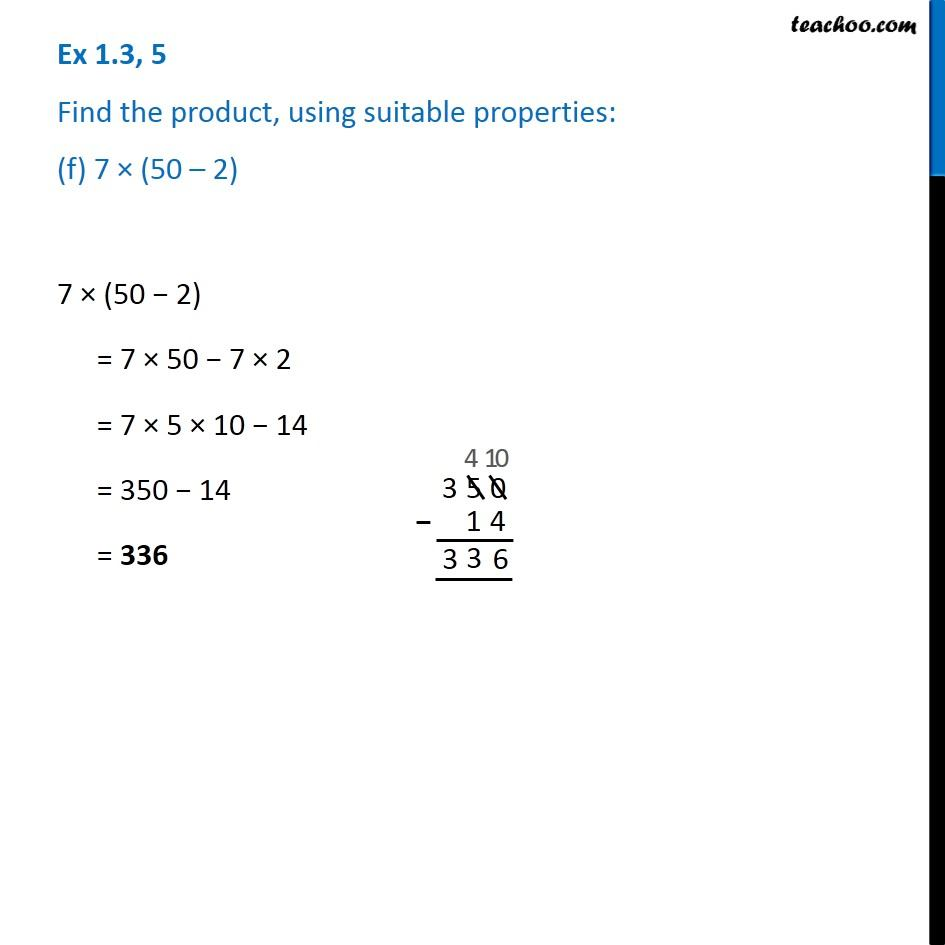1. Chapter 1 Class 7 Integers
2. Serial order wise
3. Ex 1.3

Transcript

Ex 1.3, 5 Find the product, using suitable properties: (f) 7 × (50 – 2) 7 × (50 − 2) = 7 × 50 − 7 × 2 = 7 × 5 × 10 − 14 = 350 − 14 = 336